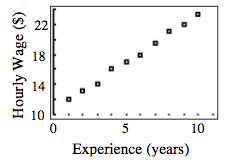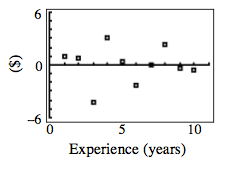### Home > INT1 > Chapter 4 > Lesson 4.2.3 > Problem4-86

4-86.

A human resources manager recorded the experience and hourly wage for a sample of $10$ technology workers.

 Experience (years) $1$ $2$ $3$ $4$ $5$ $6$ $7$ $8$ $9$ $10$ Hourly Wage ($) $12.00$ $13.25$ $14.00$ $16.00$ $17.00$ $18.00$ $19.50$ $21.00$ $22.00$ $23.25$ 1. Sketch a scatterplot showing the association between the wage and the years of experience. Describe the association. • Create a scatterplot with the $x$-axis labeled "Experience (years)" and the y-axis labeled "Hourly Wage ($)."

See answer at right. Remember to describe the association.1. Sketch the residual plot. Is a linear model appropriate?

• Refer to the Math Notes box in this lesson to review residual plots.

Label the $x$-axis "Experience (years)" and the $y$-axis "Hourly Wage (\$) as you did with the scatterplot.

See answer at right. Is the linear model appropriate?
Why or why not?1. What is the correlation coefficient? What does it tell you?

• Remember that the correlation coefficient, $r$, is a measure of how much or how little data is scattered around the LSRL.

Click the link at right for the full version of the eTool: Int1 4-86 HW eTool